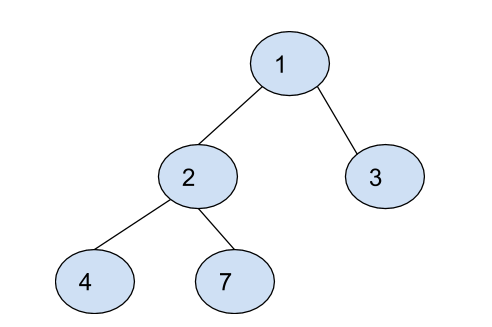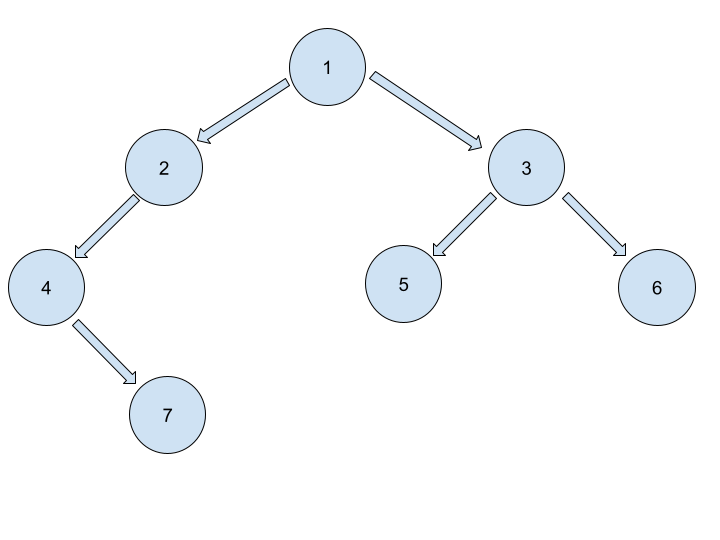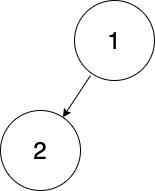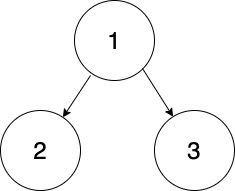11

Construct Binary Tree From Inorder and Preorder Traversal

Difficulty: EASY
Avg. time to solve
10 min
Success Rate
90%

Problem Statement

You have been given the preorder and inorder traversal of a binary tree. Your task is to construct a binary tree using the given inorder and preorder traversals.

Note:
You may assume that duplicates do not exist in the given traversals.
For example :
For the preorder sequence = [1, 2, 4, 7, 3] and the inorder sequence = [4, 2, 7, 1, 3], we get the following binary tree.Input Format:
The first line contains an integer 'T' which denotes the number of test cases or queries to be run. Then the test cases are as follows.

The first line of each test case contains an integer ‘N’ denoting the number of nodes in the binary tree.

The second line of each test case contains ‘N’ integers denoting the preorder traversal of the binary tree.

The third line of each test case contains ‘N’ integers denoting the inorder traversal of the binary tree.
Output Format:
For each test case, print the level order traversal of the constructed binary tree separated by a single-space.

For example, the output for the tree depicted in the below image would be :Level Order Traversal:
1
2 3
4 5 6
7

Explanation :
Level 1 :
The root node of the tree is 1

Level 2 :
Left child of 1 = 2
Right child of 1 = 3

Level 3 :
Left child of 2 = 4
Right child of 2 = null
Left child of 3 = 5
Right child of 3 = 6

Level 4 :
Left child of 4 = null
Right child of 4 = 7
Left child of 5 = null
Right child of 5 = null
Left child of 6 = null
Right child of 6 = null

Level 5 :
Left child of 7 = null
Right child of 7 = null
Note :
Here, if the node is null, print nothing. The above format was just to provide clarity on how the output is formed for a given tree.
The sequence will be put together in a single line separated by a single space. Hence, for the above-depicted tree, the output will be:

1 2 3 4 5 6 7

The output of each test case will be printed in a separate line.
Note :
You do not need to print anything; it has already been taken care of. You just need to return the root node of the constructed binary tree.
Constraints:
1 <= T <= 100
1 <= N <= 3000
1 <= data <= 10^4

Where ‘T’ is the number of test cases, and ‘N’ is the total number of nodes in the binary tree, and “data” is the value of the binary tree node.

Time Limit: 1sec
Follow-up:
Can you solve this in O(N) time complexity?
3
5
1 2 4 7 3
4 2 7 1 3
2
1 2
2 1
3
1 2 3
2 1 3
1 2 3 4 7
1 2
1 2 3
Explanation of Sample Input 1:
For the first test case, the tree after the construction is shown below.For the second test case, the level order of the constructed tree is [1, 2]. The tree after the construction is shown below.For the third test case, the level order of the constructed tree is [1, 2, 3]. The tree after the construction is shown below.2
3
1 2 3
3 2 1
1
7
7
Sample Output 2:
1 2 3
7Console